Courses

# Test: Motion in a Straight Line- 2

## 25 Questions MCQ Test Physics For JEE | Test: Motion in a Straight Line- 2

Description
This mock test of Test: Motion in a Straight Line- 2 for Class 11 helps you for every Class 11 entrance exam. This contains 25 Multiple Choice Questions for Class 11 Test: Motion in a Straight Line- 2 (mcq) to study with solutions a complete question bank. The solved questions answers in this Test: Motion in a Straight Line- 2 quiz give you a good mix of easy questions and tough questions. Class 11 students definitely take this Test: Motion in a Straight Line- 2 exercise for a better result in the exam. You can find other Test: Motion in a Straight Line- 2 extra questions, long questions & short questions for Class 11 on EduRev as well by searching above.
*Multiple options can be correct
QUESTION: 1

### The displacement x of a particle depend on time t as x = at2 - bt3

Solution:

Particle will return to its starting point when x = 0 by solving we get t = 0 or t = a/b
The particle will come to rest when v = 0 i.e. dx/dt = 0 which is at t = 0 or t = 2a/3b
The initial velocity of the particle = 0 but initial acceleration dv/dt (at t = 0) = 2a
F = ma and a = 2a – 6bt
Acceleration at a/3b = 0 and if a = 0 then F = 0.

*Multiple options can be correct
QUESTION: 2

### A particle has intial velocity 10 m/s. It moves due to constant retarding force along the line of velocity which produces a retardation of 5 m/s2. Then -

Solution:

The displacement of the particle is till the final velocity becomes zero. so,applying
v^2-u^2=2a s we get s=10m
Now, frm the equation s=ut - 1/2a t^2 we get that final velocity becomes zero at time t=2 sec

So the distance travelled is

s(at 3rd sec)+10=u - a/2(2n-1) [here n is 3]

after putting all the values we get

s=12.5m

*Multiple options can be correct
QUESTION: 3

### Mark the correct statements for a particle going on a straight line

Solution:

If the velocity is zero at an instant, then acceleration may not necessarily be zero at that instant. For e.g. when an object is thrown vertically upward, at highest point velocity is zero but acceleration on it due to gravity is non-zero.

*Multiple options can be correct
QUESTION: 4

A particle initially at rest is subjected to two forces. One is constant, the other is a retarding force proportion at to the particle velocity. In the subsequent motion of the particle

Solution:

This represents a case that is similar to that of a raindrop attaining terminal velocity.
Fnet = (k1 – k2v)
The acceleration wil become 0 and the velocity will be constant eventually.
Hence B and D

QUESTION: 5

In a shaping operation, the average cutting speed is ( Stroke length S, Number of strokes per minute N, Quick return ratio R)

Solution:

Time for forward stroke + Tf
Time for return stroke = Tr
R = Tr/Tf
Therefore, time for only one cutting stroke(T) = 1/N x Tf/(Tf + Tr)
Average cutting speed = S/T = SN(Tf + Tr)/Tf = SN(1 + R).

*Multiple options can be correct
QUESTION: 6

Which of the following statements are true for a moving body?

Solution:

v=change in displacement/change in time ​ and
a=change in velocity/change in time ​
Speed is the magnitude of velocity.
There can be a case where there is change in direction but not in magnitude.

*Multiple options can be correct
QUESTION: 7

Let v and a denote the velocity and acceleration respectively of a body

Solution:

A can be zero when velocity and vice-versa you can get that when you see a pendulum at mean a = 0 but v = max and at extreme a = max when v = 0. Also a “can” be zero when v is zero.

*Multiple options can be correct
QUESTION: 8

A tennis ball is thrown straight up and caught at the same height. Which of the following can describe the motion of the ball when it reaches the apex?

Solution:

When the ball is at its peak possible position, it's velocity remains Zero. Unfortunately, this moment of zero velocity is hardly visible, since it occurs in a very very short amount of time.
Now if you ask about the acceleration at the peak possible position, it turns out to be very interesting. The acceleration remains 9.81 ms^-2. In fact it is one of the very rare situations where the body is accelerated, still there is an absolute zero velocity.

*Multiple options can be correct
QUESTION: 9

Velocity-time graph for a car is semicircle as shown here. Which of the following is correct :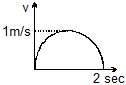Solution:

Option A: Acceleration varies as slope of this graph; circular motion does not have the acceleration shown by this graph.
Option B: Acceleration is zero at t=1s, when slope is 0.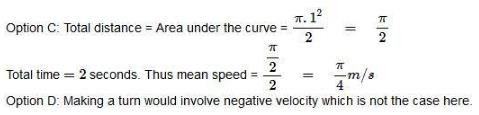*Multiple options can be correct
QUESTION: 10

The figure shows the velocity (v) of a particle plotted against time (t)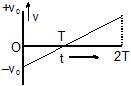Solution:
1. As it crossed the x axis so the direction changed
2. The slope of v-t graph gives acceleration so it is constant
3. The net areas below and above x-axis are equal so the displacement is zero
4. Speed is a scalar quantity hence the initial and final speeds are same
*Multiple options can be correct
QUESTION: 11

A block is thrown with a velocity of 2 ms-1 (relative to ground) on a belt, which is moving with velocity 4 ms-1 in opposite direction of the initial velocity of block. If the block stops slipping on the belt after 4 sec of the throwing then choose the correct statements(s)

Solution:

ATQ: u = 2 m/s, v = -4 m/s, so,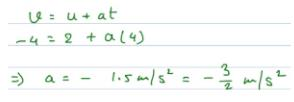Now, to find the time at which the displacement is zero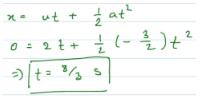Now, to find the displacement at time t = 4 secs wrt the ground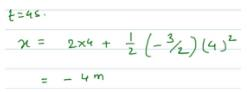And the displacement at time t = 4 secs wrt the belt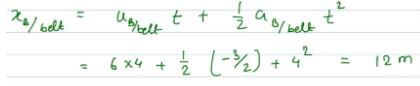*Multiple options can be correct
QUESTION: 12

A particle moves with constant speed v along a regular hexagon ABCDEF in the same order. Then the magnitude of the average velocity for its motion from A to -

Solution: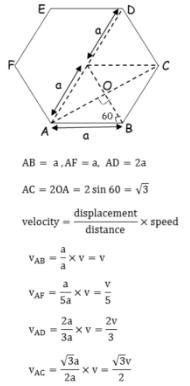*Multiple options can be correct
QUESTION: 13

An observer moves with a constant speed along the line joining two stationary objects. He will observe that the two objects

Solution:

Given constant speed this question so speed is same, since it moved on a straight line so, velocity is same and in the same direction.

*Multiple options can be correct
QUESTION: 14

A man on a rectilinearly moving cart, facing the direction of motion, throws a ball straight up with respect to himself

Solution:

The ball will return to him if the cart moves with a constant velocity because the ball will travel the same amount of distance as the cart in between the time interval when it moves up and returns to the same horizontal level. But it will fall behind if the cart has acceleration because then the amount of distance moved by the cart will be more.

*Multiple options can be correct
QUESTION: 15

A projectile of mass 1 kg is projected with a velocity of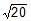m/s such that it strikes on the same level as the point of projection at a distance of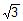m. Which of the following options are incorrect.

Solution:

R=u2sin2θ=√3
(√20)2sin2θ/10=√3
sin2θ=√3/2
2θ=60o,120o
(A)θ=30o
μ=u2sin2θ/2g=(√20)2sin230/2x10=0.25
θ=60°
μ=(√20)2sin230/2x10=3x0.25
(B) Umin=ucosθ=√20cos30°=√5m/s
(C) T=2usinθ/g  For θ=30°
��=2x√20sin260°/10=√3/5
(D)Umax=mgh=1x10x0.25=2.5J

*Multiple options can be correct
QUESTION: 16

Choose the correct alternative (s)

Solution:

Let A be the angle of projection and u be the velocity of projection.
(A) Maximum height = u²/2g
Range = (u²sin 2A)/g
Maximum range (at A = π/4) = u²/g
So, max range = 2 × max height

(B) Max Height = u²sin²A/2g
Range = (2u²sin A cos A)/g
Range = n × Max Height
(2u²sin A cos A)/g = n × (u²sin²A)/2g
4sin A cos A = n × sin²A
tan A = 4/n

(C) The displacement in y-direction is zero.
0 = (u sin A)t - gt²/2
t = 2u sin A/g
R = (2u²sin A cos A)/g
2u²sin A cos A = gR
Multiply by 2 tan A
4u²sin²A = 2gR tan A
(2u sin A)² = 2gR tan A
g²t²= 2gR tan A
gt²= 2R tan A

(D) For vertical motion, t = 2u/g
For projectile of, T = 2u sin A/g
If T = t it means sin A = 1 => A = π/2
So, both are vertical motions and hence thier maximum heights will be same (or) their ratio is 1:1

QUESTION: 17

The v-t graph of the particle is correctly shown by

Solution:

Explanation : Since the x−t graph is parabola which is of second degree the v−t graph must be a straight line which is of first degree.

The slope of the graph in the period 0≤t<T is positive and decreasing which implies that the velocity is also positive and decreasing in this period.

The slope of the graph at t=T is 0 which implies that the velocity is also 0 at this time.

The slope of the graph in the period T≤t≤2T is negative and decreasing which implies that the velocity is also negative and decreasing in this period.

The only graph which satisfies the above conditions is graph b.

QUESTION: 18

The a-t graph of the particle is correctly shown by

Solution:

The acceleration of the particle moving in straight line is given as, a=d2x​/dt2
Geometrically it is the concavity of the x−t graph. If the graph is concave upward then the double derivative is positive. If the graph is concave downwards then the double derivative is negative.Consider the provided x−t graph,
Since the x−t graph is a parabola which of second degree the a−t graph must be a constant line which is of zero degree. Since the parabola is concave downwards the acceleration must be negative.
The only graph which satisfies the above conditions is graph D.
Hence, option D is correct.

QUESTION: 19

The speed-time graph of the particle is correctly shown by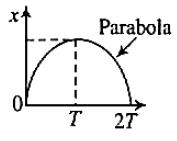Solution:

The speed of the particle moving in straight line is always positive and given as, s=∣dx/dt​∣

Geometrically it is the modulus of the slope of the x−t graph.

Consider the provided x−t graph,

Since the x−t graph is a parabola which is of second degree the s−t graph must be a straight line which is of first degree.

The slope of the graph in the period 0≤t<T is positive and decreasing which implies that the speed is positive and decreasing in this period.

The slope of the graph at t=T is 0 which implies that the speed is also 0 at this time.

The slope of the graph in the period T≤t<2T is negative and decreasing which implies that the speed is positive and decreasing in this period.

The only graph which satisfies the above conditions is graph C.QUESTION: 20

The figure shows a velocity-time graph of a particle moving along a straight line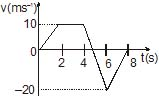Choose the incorrect statement. The particle comes to rest at

Solution:

The particle comes to rest (v = 0) at two instants. It comes to rest first time at somewhere between 4th second and 6th second. At t = 8 second it again comes to rest.
We will find when the particle comes to rest for the first time. Between 4th and 6th second (time interval of 2 seconds) the particle changes it's velocity from 10 m/s to -20 m/s. So, the acceleration on the particle during this part of motion is,
a = (-20 - 10)/2 = -15 seconds
The particle comes to rest when v = 0
v - u = at
0 - 10 = (-15)t
⇒ t = 1.5 seconds
So the particle come to rest at (4 + 1.5)th = 5.5 th second and 8th second

QUESTION: 21

A train covers 60 miles between 2 p.m. and 4 p.m. How fast was it going at 3 p.m.?

Solution:

The speed is traveled distance (60 miles) divided by traveled time (4pm – 2pm = 2hours):
60 miles/ 2 hours = 30 mph

QUESTION: 22

The figure shows a velocity-time graph of a particle moving along a straight lineIf the particle starts from the position x0 = -15 m, then its position at t = 2s will be

Solution:

Displacement of the particle in 2 seconds is the area under v-t graph till 2 seconds,
s = (1/2)(10)(2) = 10 m
Net displacement = -15 + 10 = -5 m

QUESTION: 23

The figure shows a velocity-time graph of a particle moving along a straight lineThe maximum of displacement of the particle is

Solution:

The area under the v-t graph gives displacement. The maximum displacement is when the particle velocity becomes zero and it changes its direction. Since we don't know when the particles crosses x-axis (v = 0) we will first find displacement till 4 seconds
S = (1/2)(10)(2) + (10)(2) = 30 m
The particle velocity changes from 10 m/s to - 20 m/s in next 2 seconds. So it's acceleration during this part of motion is,
a = (-20 - 10)/2 = - 15 m/s²
Distance travelled till velocity becomes zero can be calculated by using the formula v² - u² = 2as
0 - (10)² = 2(-15)s
=> s = 100/30 = 3.33 m
Therefore the maximum displacement is,
d = 30 + 3.3 = 33.3 m

QUESTION: 24

The figure shows a velocity-time graph of a particle moving along a straight lineThe total distance travelled by the particle is

Solution:

Total distance is the entire area under the curve which is area of trapezium + area of triangle.
Trapezium = |½ x 10 x (5 + 2)| = 35
Triangle = |½ x -20 x 2| = 30
Distance = 65m

QUESTION: 25

The figure shows a velocity-time graph of a particle moving along a straight lineThe correct displacement-time graph of the particle is shown as

Solution:

Displacement is said to the shortest path covered by a body or object. Hence, when the velocity is positive the object moves in one direction and when its negative then in the opposite direction thus making the displacement first increase then decrease.